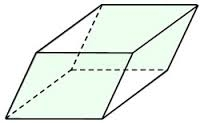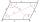# The diamond

The diamond has an area S = 120 cm2, the ratio of the length of its diagonals is e: f = 5: 12. Find the lengths of the side and the height of this diamond.

a =  13 cm
h =  9.2308 cm

### Step-by-step explanation:Did you find an error or inaccuracy? Feel free to write us. Thank you!Tips to related online calculators
Check out our ratio calculator.
Do you want to convert length units?
Pythagorean theorem is the base for the right triangle calculator.

#### You need to know the following knowledge to solve this word math problem:

We encourage you to watch this tutorial video on this math problem:

## Related math problems and questions:

• Diamond diagonalsFind the diamond diagonal's lengths if the area is 156 cm2 and side is 13 cm long.
• Diamond diagonalsCalculate the diamonds' diagonals lengths if the diamond area is 156 cm square, and the side length is 13 cm.
• Diamond diagonalsCalculate the diamond's diagonal lengths if its content is 156 cm2 and the side length is 13 cm.
• Rhombus and diagonalsThe rhombus area is 150 cm2, and the ratio of the diagonals is 3:4. Calculate the length of its height.
• Diagonals of the rhombusHow long are the diagonals e, f in the diamond, if its side is 5 cm long and its area is 20 cm2?
• Rhombus and diagonalsThe lengths of the diamond diagonals are e = 48cm, f = 20cm. Calculate the length of its sides.
• The diamondThe diamond has sides of 35 cm and the diagonals are in a ratio of 1: 2. Calculate the lengths of the diagonals.
• Two diagonalsThe diagonals of the diamond EFGH have lengths in the ratio 1: 2. What is the circumference of a rhombus if the longer of the diagonals is 8 cm long?
• Diagonals of a rhombus 2One diagonal of a rhombus is greater than the other by 4 cm. If the area of the rhombus is 96 cm2, find the side of the rhombus.
• Rhombus diagonalsIn the rhombus ABCD are given the sizes of diagonals e = 24 cm; f = 10 cm. Calculate the side length of the diamond and the size of the angles, calculate the content of the diamond
• Diagonals of a rhombusOne of the diagonals of a rhombus is twice as the other. If the sum of the lengths of the diagonals is 24, find the area of the rhombus.
• Diagonals in the diamondThe length of one diagonal in a diamond is 24 cm greater than the length of the second diagonal, and the diamond area is 50 m2. Determine the sizes of the diagonals.
• DiagonalsGiven a rhombus ABCD with a diagonalsl length of 8 cm and 12 cm. Calculate the side length and content of the rhombus.
• Rectangle diagonalsIt is given a rectangle with an area of 24 cm2 a circumference of 20 cm. The length of one side is 2 cm larger than the length of the second side. Calculate the length of the diagonal. Length and width are yet expressed in natural numbers.
• RhombusThe rhombus has diagonal lengths of 4.2cm and 3.4cm. Calculate the length of the sides of the rhombus and its height
• Two diagonalsThe rhombus has a side length of 12 cm and a length of one diagonal of 21 cm. What is the length of the second diagonal?
• Diamond and anglesFind the area of a diamond with a side of 5 cm if you know that the internal angles in the diamond are 60° and 120°.